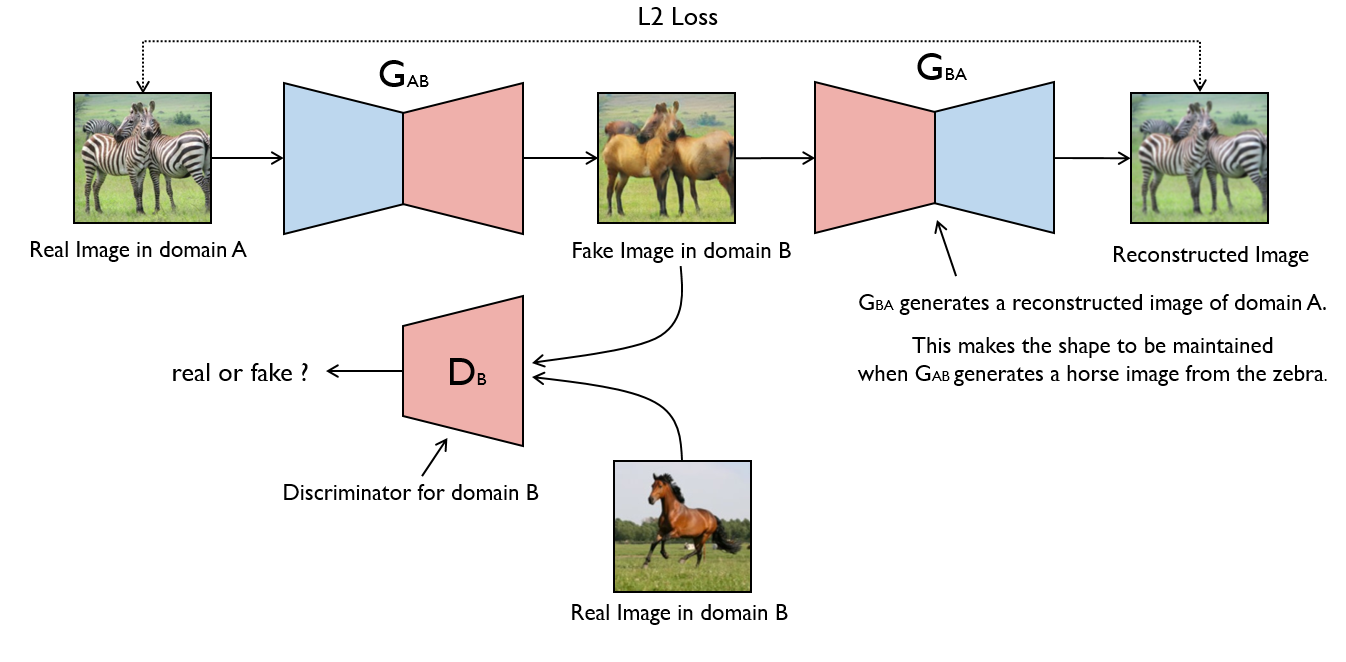# Introduction to CycleGAN

Generative Adversarial Network or in short GAN, is an unsupervised machine learning task that involves automatically discovering and learning the regularities or patterns in input data to generate new data synthetically.CycleGAN Architecture Image Courtesy : https://modelzoo.co/model/mnist-svhn-transfer

# Implementation

1. Input pipeline and Normalization
2. Model Building
• Generator Model Design
• Discriminator Model Design
• Loss Functions

# 1. Input Pipeline

The reference T1 and T2 images are loaded with a specified image size. Image size of (256,256) with grayscale mode was imported into the tensor Batchdataset.

# 2. Model Building

The CycleGAN model consists of three important modules

1. Generator
2. Discriminator
3. Loss functions

# Generator Design

The Generator is the module which actually generates the synthetic image starting with a random noise signal.

• Generator_g learns to transform image from T1 to T2
• Generator_f learns to transform image from T2 to T1

# Discriminator Design

The major task of discriminator module is to classify the synthetic image from the generator as real or fake.

• Discriminator_X learns to differentiate between image X and generated image X.
• Discriminator_Y learns to differentiate between image Y and generated image Y

# Loss Functions

1. Generator loss : It is a binary cross entropy loss of the generated images and an array of ones
2. Discrimantor loss Consists of 2 inputs
— real_loss is a binary cross entropy loss of the real images and an array of ones(since these are the real images)
— generated_loss is a sigmoid cross entropy loss of the generated images and an array of zeros(since these are the fake images)
— total_loss is the sum of real_loss and the generated_loss
3. Cycle Consistency Loss — Cycle consistency means the result should be close to the original input. If T1 image is translated to T2 image, and then translates it back from T2 to T1, then the resulting image should be the same as the original image
4. Identity Loss — If the image is fed to the generator, it should yield the real image or something close to image.

# 3. Training

The training consists of four broad steps

1. Get the predictions — The generator and discriminator modules are used to get the predictions
2. Calculate the loss — Various loss functions defined above are used to calculate the losses
3. Calculate the gradients using backpropagation
4. Apply the gradients to the optimizer — Adam optimizer as defined in the original paper is used for optimization

# 4. Final Predictions

Below are the predictions based on the trained model

# References and Links

1. Unpaired Image-to-Image Translation using Cycle-Consistent Adversarial Networks by Jun-Yan Zhu, Taesung Park, Phillip Isola, Alexei A. Efros
https://arxiv.org/abs/1703.10593
2. Generative Adversarial Networks for Image-to-Image Translation on Street View and MR Images by Simon Karlsson & Per Welander
http://liu.diva-portal.org/smash/get/diva2:1216606/FULLTEXT01.pdf
3. https://www.tensorflow.org/tutorials/generative/cyclegan
4. https://machinelearningmastery.com/cyclegan-tutorial-with-keras/

--

--